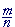S k i l l
i n
A L G E B R A

26

# Radicals:  Rational and Irrational Numbers

The square numbers

2nd level:

Equations  (x + a)² = b

HERE ARE THE FIRST TEN square numbers  and their roots:

 Square numbers 1 4 9 16 25 36 49 64 81 100 Square roots 1 2 3 4 5 6 7 8 9 10

We write, for example,=  5.

"The square root of 25 is 5."

This markis called the radical sign (after the Latin radix = root). The number under the radical sign is called the radicand.  In the example, 25 is the radicand.

Problem 1.   Evaluate the following.

Do the problem yourself first!

 a)=  8 b)=  12 c)=  20 d)=  17 e)=  1 f)= 79

Example 1.   Evaluate.

Solution.= 13.

For, 13· 13 is a square number.  And the square root of 13· 13 is 13If a is any whole number, then a· a is a square number, andProblem 2.   Evaluate the following.

 a)=  28. b)=  135.
 c)=  2· 3· 5 = 30.

We can state the following theorem:

A square number times a square number is itself a square number.

For example,

36· 81 = 6· 6· 9· 9 = 6· 9· 6· 9 = 54· 54

Problem 3.   Without multiplying the given square numbers, each product of square numbers is equal to what square number?

a)  25· 64 = 5· 8· 5· 8 = 40· 40

b)  16· 49 = 4· 7· 4· 7 = 28· 28

c)  4· 9· 25 = 2· 3· 5· 2· 3· 5 = 30· 30

Rational and irrational numbers

The rational numbers are the everyday numbers of arithmetic:  the whole numbers, fractions, mixed numbers, and decimals; together with their negative images.  A rational number has the same ratio to 1 as two natural numbers.

That is what a rational number is. As for what it looks like, it can take the form, where a and b are integers (b ≠ 0).

Problem 4.   Which of the following numbers are rational?

 1 −6 3½ 45 − 13 5 0 7.38609

All of them.

At this point, the student might wonder, What is a number that is not rational?

An example of such a number is("Square root of 2").is not a number of arithmetic.  We cannot name any whole number, any fraction

 or any decimal whose square is 2. 75 is close, because
 75 · 75 = 4925

-- which is almost 2.

But to prove that there is no rational number whose square is 2, then suppose there were. Then we could express it as a fractionin lowest terms. That is, suppose·= 2.

But that is impossible.  Sinceis in lowest terms, then m and n have no common divisors except 1.  Therefore, m· m and n· n also have no common divisors -- they are relatively prime -- and it will be impossible to divide n· n into m· m and get 2.

There is no rational number whose square is 2.  Therefore we callan irrational number.

Question.   The square roots of which natural numbers are rational?

Answer.   Only the square roots of square numbers.= 1  RationalIrrationalIrrational= 2  Rational,,,Irrational= 3  Rational

And so on.

The square roots of the square numbers are the only square roots that we can name.

The existence of irrationals was first realized by Pythagoras in the 6th century B.C.  He called them "without a name."  For if we ask, " How much is? -- we cannot say.  We can only call it, "Square root of 2."

Problem 5.   Say the name of each number.

 a)Square root of 3. b)Square root of 8. c)3.
 d)25 e)Square root of 10

As for the decimal representation of both irrational and rational numbers, see Topic 2 of Precalculus.

An equation  x² = a, and the principal square root

Example 2.   Solve this equation:

 x² = 25. Solution. x = 5  or  −5, because (−5)² = 25, also. In other words, x =or  −.

We say however that the positive value 5 is the principal square root. That is, we say that "the square root of 25" is 5.= 5.

As for −5, it is "the negative of the square root of 25."= −5.

Thus the symbolrefers to one non-negative number.

Example 3.   Solve this equation:

 x² = 10. Solution. x =or  −.

Always, if an equation looks like this,

 x² = a, then the solution will look like this: x =or  −. We often use the double sign  ± ("plus or minus")  and write: x = ±.

Problem 6.   Solve for x.

 a) x² = 9  implies x = ±3 b) x² = 144  implies x = ±12 c) x² = 5  implies x = ±d) x² = 3  implies x = ±e) x² = a − b  implies x = ±2nd Level